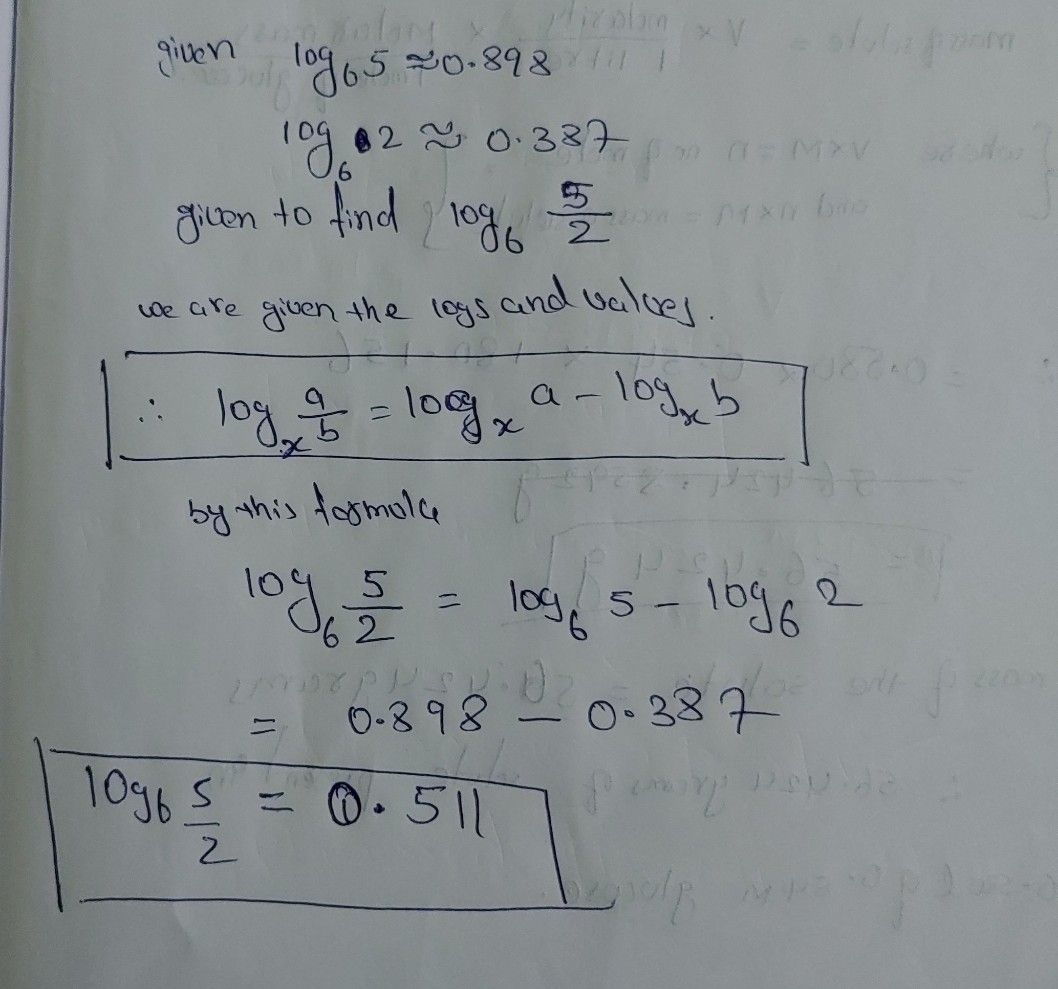Symbol
ProblemUse $log _{6}5=0.898$ and $log _{6}2=0.387$ to evaluate $log _{6}\dfrac {5} {2}$ WORK REQUIRED $\left(3$ pts.) $\right)$ $a.$ $2.322$ $m.$ $0.511$ c. $0.613$ d. $0.431$